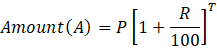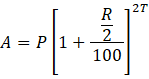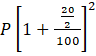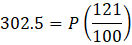# A person deposits a certain amount of money at the interest rate of 20% per annum compounded twice a year. If the person gets Rs. 302.50 after 1 year, then what will be the amount he invested in the starting?

2019-08-27 | Team PendulumEdu

Options:

A. Rs. 294

B. Rs. 240

C. Rs. 250

D. Rs. 280

Solution:

Amount (A) obtained on a principal amount (P), which is subjected to a compound interest rate (R) for a time period of (T) is given asGiven

Rate (R) = 20% per annum compounded twice a year

Time (T) = 1 year

Amount = Rs. 302.5

Let the principal amount he/she invested in the staring be Rs. P

For Periodic compounding within a year

Effective rate is taken as (1/n)th  times of rate given and effective time is taken as nth times of the time period given

Rate of interest compounded twice a year means Rate of interest compounded half yearly

So, Effective rate = 20/2 = 10%

And, Effective time period = 2*1 = 2

Now, Actual amount obtained because of compounding will beP = 2.5*100 = Rs. 250

Hence, (C) is the correct answer.

Such type of question is asked in various competitive exams like SSC CGL, SSC MTS, SSC CPO, SSC CHSL, RRB JE, RRB NTPC, RRB GROUP D, RRB OFFICER SCALE-I, IBPS PO, IBPS SO, RRB Office Assistant, IBPS Clerk, RBI Assistant, IBPS RRB OFFICER SCALE 2&3, etc. Try and attempt free mock tests at PendulumEdu and learn new techniques to solve such questions in less time. Also, PendulumEdu offers one-to-one session to clear all exam related doubts.0